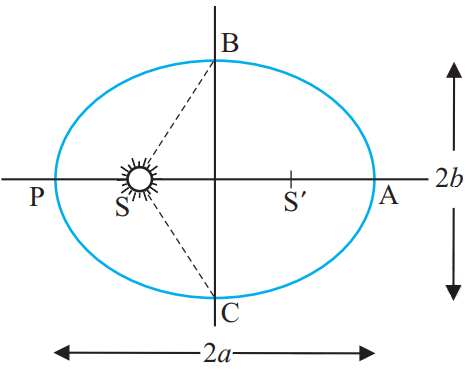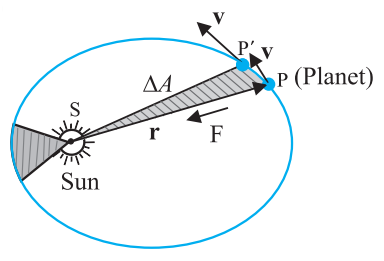# Planetary Formulas

## Kepler’s Laws

Kepler’s Laws for planetary motion is found by Johannes Kepler and is stated as below.

Kepler’s first law: Law of OrbitsKepler’s first law states that “All planets move around the sun in elliptical orbits with the sun at one focus”.

Explanation: An ellipse traced out by a planet around the sun. The closest point is P and the farthest point is A, P is called the perihelion and A the aphelion. The semimajor axis is half the distance AP.

Kepler’s second law states that “The line joining a planet to the Sun sweeps out equal areas in equal interval of time”.Explanation:

P is the planet that moves around the sun in an elliptical orbit

∆A is the area swept

∆t is the time interval

Kepler’s Third Law states that “The square of the time period of the planet is directly proportional to the cube of the semimajor axis of its orbit”

$$\begin{array}{l}P^{2}\alpha a^{3}\end{array}$$

Kepler’s third law is generalised after applying Newton’s Law of Gravity and laws of Motion.

$$\begin{array}{l}P^{2}=\frac{4\pi^{2}}{G(M1+M2)}(a^{3})\end{array}$$

Where,

M1 and M2 are the masses of the orbiting objects

### Orbital Velocity Formula

Orbital velocity formula is used to calculate the orbital velocity of planet with mass M and radius R.

$$\begin{array}{l}V_{orbit}=\sqrt{\frac{GM}{R}}\end{array}$$

Where,

G = Gravitational constant = 6.67 × 10-11 m3/s2 kg

For more planetary formulas visit BYJU’S app!!